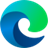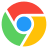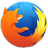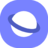# rotateX() CSS Function

### Description

The CSS `rotateX()` function is used to rotate an element around the horizontal axis (x-axis). It is part of the CSS `transform` property, which allows you to apply various transformations to elements, such as scaling, rotation, and translation.

To use the `rotateX()` function, you simply need to specify the angle of rotation in the function's parentheses. The angle can be specified in degrees, gradians, radians, or turns. A positive angle will rotate the element clockwise, while a negative angle will rotate the element counter-clockwise.

For example, the following CSS code will rotate an element by 30 degrees around the horizontal axis:

``````element {
transform: rotateX(30deg);
}
``````

You can also use the `rotateX()` function in combination with other CSS transform functions to create more complex transformations. For example, the following CSS code will rotate an element by 30 degrees around the horizontal axis and then scale it by 2:

``````element {
transform: rotateX(30deg) scale(2);
}
``````

The `rotateX()` function is a powerful tool that can be used to create a variety of visual effects. It is commonly used to rotate images, text, and other elements, such as 3D models.

Here are some examples of how the `rotateX()` function can be used:

• To rotate an image of a card so that it appears to be flipping over:
``````img {
transform: rotateX(180deg);
}
``````
• To create a 3D carousel effect:
``````.carousel-item {
transform: rotateX(45deg);
}
``````
• To create a flip-up animation for text:
``````h1 {
transform: rotateX(-90deg);
transition: transform 0.5s ease-in-out;
}

h1:hover {
transform: rotateX(0deg);
}
``````

The `rotateX()` function is a versatile tool that can be used to create a variety of creative effects. With a little practice, you can use it to add depth and movement to your web pages and applications.

### Syntax

`transform: rotateX(<angle>);`

### Values

• <angle>A positive value rotates the element clockwise, a negative value rotates the element counter clockwise.

### Example

``````<div class="normal rotated">Rotated</div>
<div class="normal">Not rotated</div>``````
``````.normal {
margin: 20px;
width: 120px;
background: #546D62;
color: white;
font-family: sans-serif;
}
.rotated {
transform: rotateX(45deg);
}``````

### Browser Support

The following table will show you the current browser support for the CSS `rotateX()` function.

 Desktop12 1 3.5 10.1 3.5
 Tablets / Mobile18 4 11 3.2 1 2

Last updated by CSSPortal on: 7th October 2023